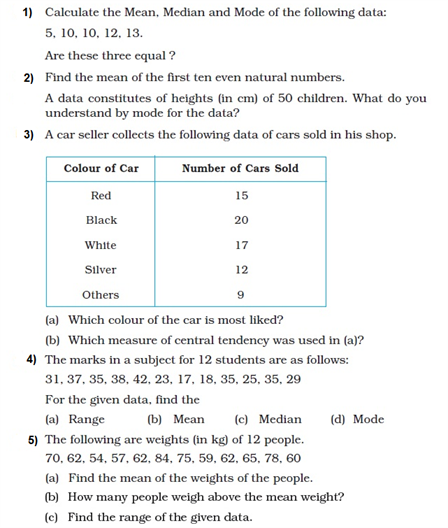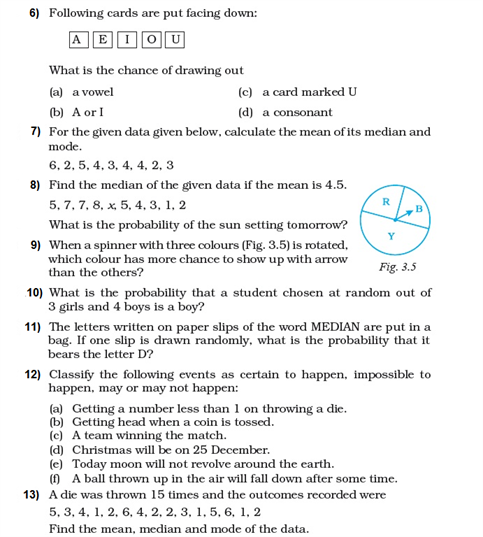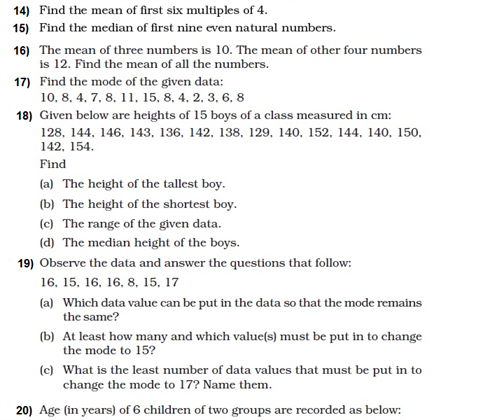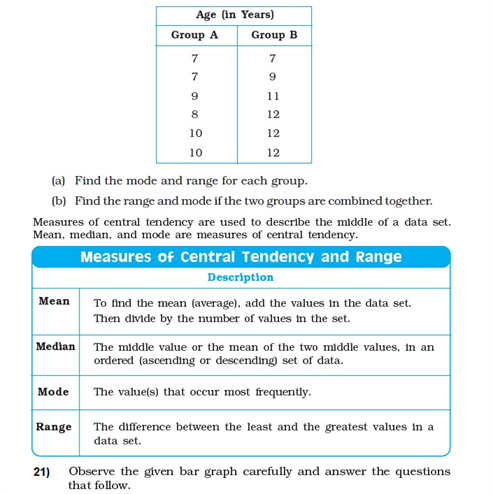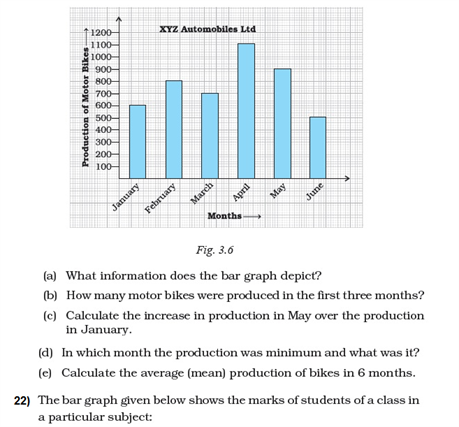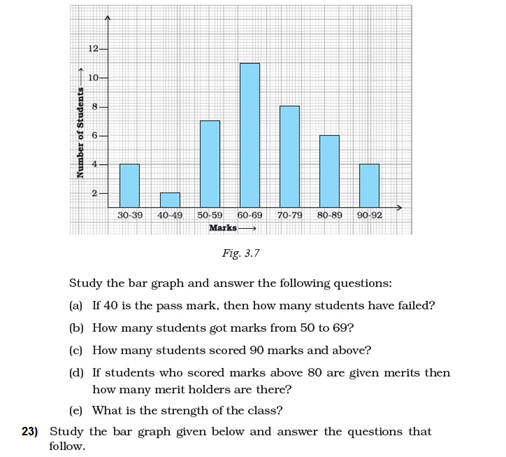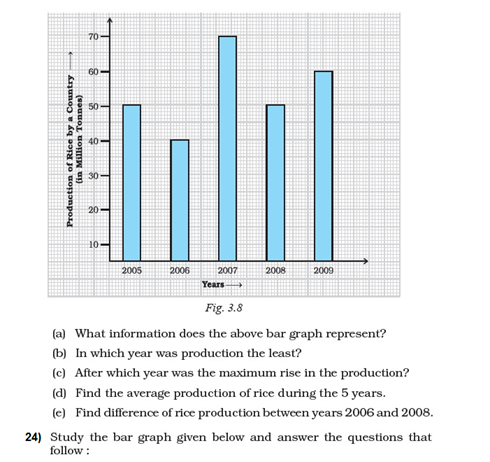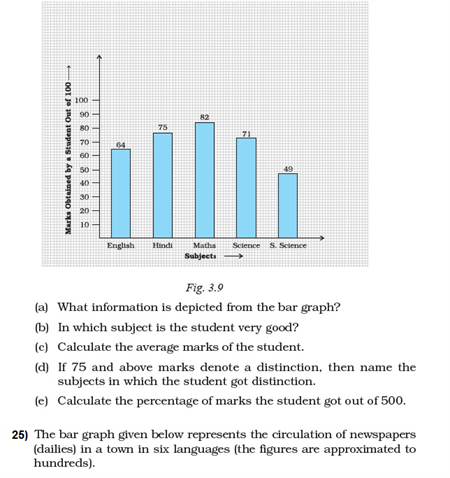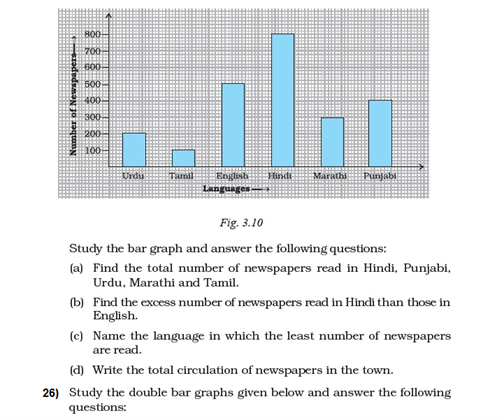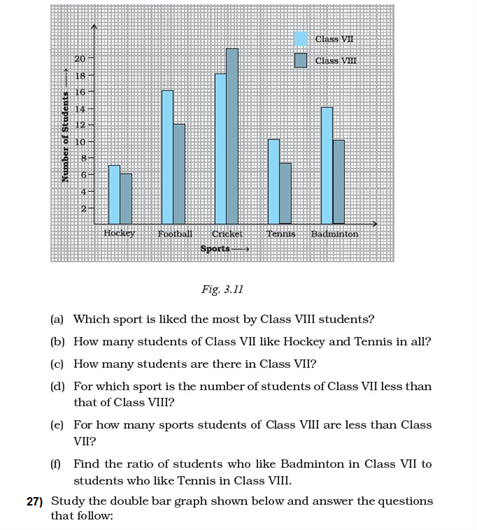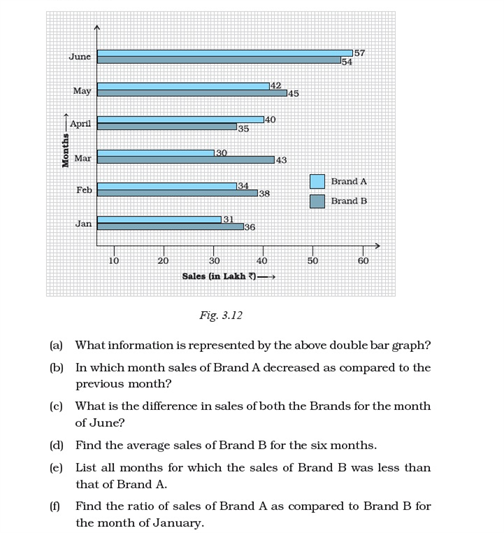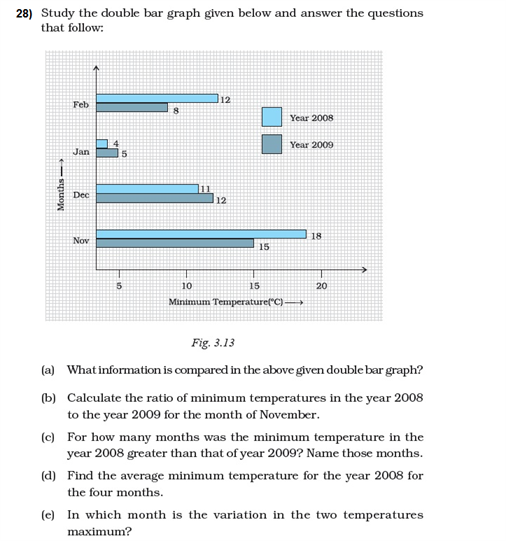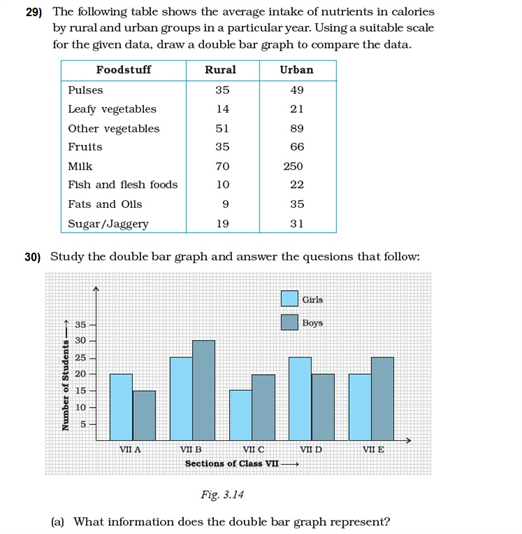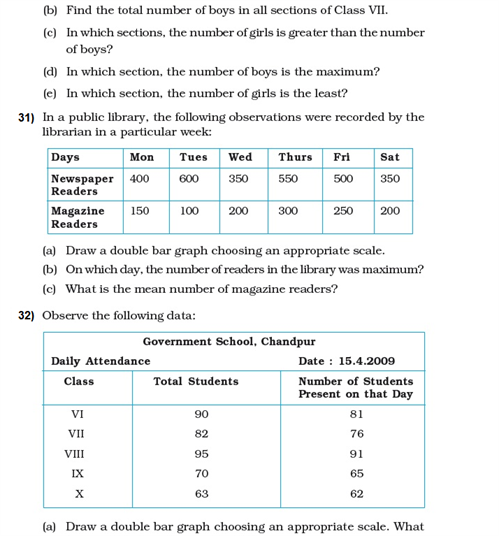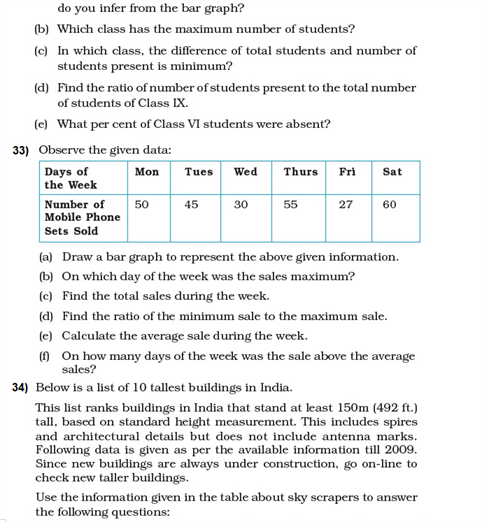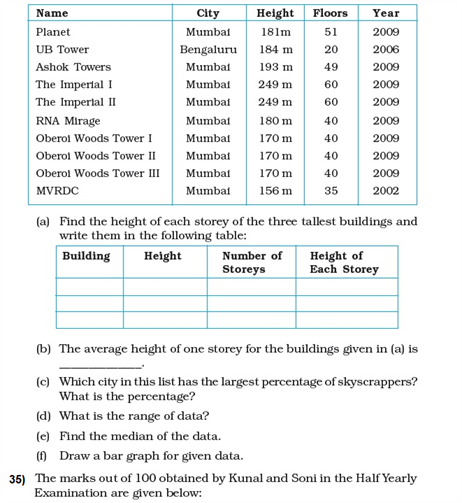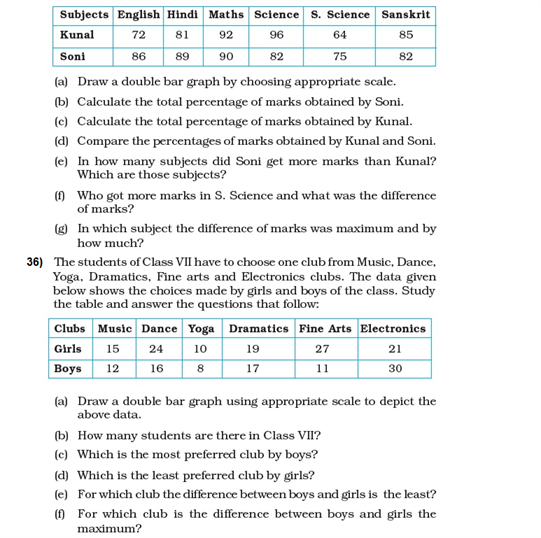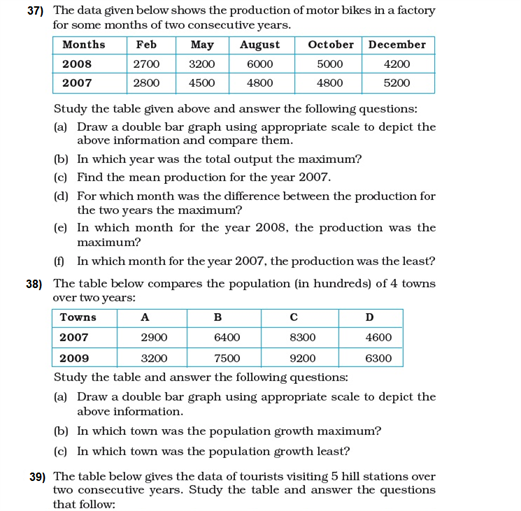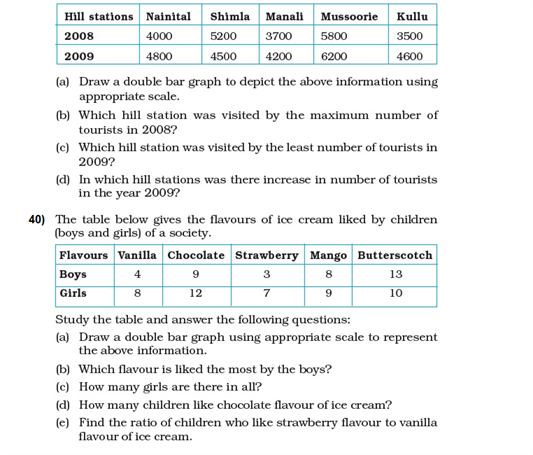# Important Questions Class 8 Maths Chapter 5 Data Handling

Important data handling questions for class 8 maths chapter 5 are provided here to help the students get completely acquainted with the concepts. These questions from class 8 data handling topic (NCERT) given require the students to have a deeper understanding of the concepts and thus, will help to develop problem-solving skills.

## Important Data Handling Questions for Class 8 (Chapter 5)

The data handling questions also include questions from “chance and probability” as the concepts are included in the NCERT class 8 maths book. Some of the most important data handling and chance and probability questions are given below.

### 1. Answer the following questions:

(i) What is the total angle at the centre of a pie chart?

(ii) What does the height and width of rectangles in a histogram represent?

(iii) Is tossing a coin a random experiment?

Solution:

(i) 360°

(ii) The height represents the number of times the values within an interval occurred and the width represents the length of the interval covered by the bar.

(iii) Yes, tossing a coin is a random experiment. To recall, a random experiment is an experiment or a process for which the outcome cannot be predicted with certainty.

2. A group of students were asked about their favourite superhero. The responses were as follows:

Batman, Superman, Ironman, Spiderman, Superman, Batman, Spiderman, Superman, Spiderman, Batman, Ironman, Batman, Ironman, Ironman, Superman, Batman, Spiderman, Batman, Ironman, Superman, Ironman, Batman.

(i) How many students like Ironman?

Solution:

Ironman is liked by 6 students.

(ii) Which is the most liked superhero?

Solution:

From the table,

Number of students like

Batman = 7

Superman = 5

Ironman = 6

Spiderman = 4

So, the most liked superhero is Batman.

(iii) Draw a frequency distribution table from the data.

Solution:

Frequency distribution table will include how often a superhero’s name is repeated.

 Superhero Frequency Batman 7 Superman 5 Ironman 6 Spiderman 4

3. Calculate the probability of picking a prime number from a lot containing the first 100 natural numbers.

Solution:

Number of elements in the sample space = 100

Total number of prime numbers = 25 (2, 3, 5, 7, 11, 13, 17, 19, 23, 29, 31, 37 41, 43, 47, 53, 59, 61, 67, 71, 73, 79, 83, 89 and 97)

So, the probability of picking a prime number from the lot = 25/100 =¼

4. A student scored the following marks in six subjects:

36, 35, 50, 46, 60, 55

Calculate the mean marks scored by him.

Solution:

Mean = sum of data points/ total number of data

So, Mean = (36 + 35 + 50 + 46 + 60 + 55)/6 = 282/6 = 47

5. A bag contains 10 red balls and 8 white balls. Find the probability of picking one white ball.

Solution:

Total number of white balls = 8

Total number of red balls = 10

So, total number of balls = 10 + 8 = 18

Here, the total favourable outcome will be the total number of white balls i.e. 8

Now,

P(The ball drawn is white) = (Number of favourable cases)/ (Total number of cases)

= 8/18 = 4/9

### More Questions From Class 8 Chapter 8 Comparing Quantities